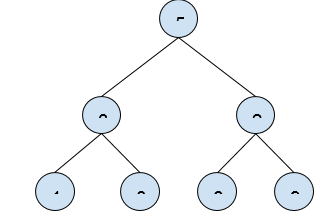# Find maximum among all right nodes in Binary Tree in C++

In this problem, we are given a Binary Tree. Our task is to find maximum among all right nodes in Binary Tree.

Problem Description: Here, we need to find the maximum value amongst all right child nodes of the binary Tree.

Let’s take an example to understand the problem,

Input:Output: 9

Explanation:

All right nodes are: {2, 8, 9}. Maximum of them is 9.

## Solution Approach

To solve the problem, we need to traverse the tree and check if its right child exists. If it exists, compare with maxRight element and replace if it is greater.

## Example

Live Demo

#include <iostream>
using namespace std;

struct Node {
int data;
struct Node *left, *right;
};

Node* newNode(int data) {

Node* temp = new Node;
temp->data = data;
temp->left = temp->right = NULL;
return temp;
}

int findMaxRightNode(Node* root) {

int maxRight = -100;

if (root == NULL)
return -1;

if (root->right != NULL)
maxRight = root->right->data;

return max( findMaxRightNode(root->right), max(maxRight, findMaxRightNode(root->left) ) );
}

int main() {

Node* root = newNode(5);
root->left = newNode(3);
root->right = newNode(2);
root->left->left = newNode(1);
root->left->right = newNode(8);
root->right->left = newNode(6);
root->right->right = newNode(9);

cout<<"The maximum among all right nodes in Binary Tree is "<< findMaxRightNode(root);

return 0;
}

## Output

The maximum among all right nodes in Binary Tree is 9

Updated on: 25-Jan-2021

38 Views